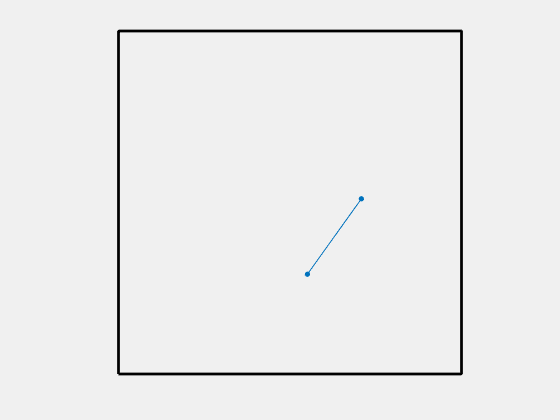# How Far Apart Are Two Random Points in a Square?

How far apart can you expect two points chosen at random in the unit square to be? I found this problem on the YouTube channel maintained by Presh Talwalkar, Mind Your Decisions. He correctly calls it a very hard puzzle. At first, I guessed the answer might be $1/2$. But the correct answer is more interesting than that.### Contents

#### Simulation

Let's do a Monte Carlo simulation to get a numerical estimate. Sampling one million pairs of points doesn't take much time.

   n = 1000000;
sum = 0;
rng(0)
for k = 1:n
x = rand(1,2);
y = rand(1,2);
delta = norm(x-y);
sum = sum + delta;
end
format short
delta = sum/n

delta =
0.5214


It turns out that this run of the simulation generates a result that is accurate to the four digit precision of format short. But can we find the exact value?

The expected distance, $\delta$, can be expressed as this quadruple integral, but the Symbolic Toolbox cannot find a closed form.

$$\delta = \int_0^1 \int_0^1 \int_0^1 \int_0^1 \! \sqrt{(x_1-y_1)^2 + (x_2-y_2)^2} \ \mathrm{d}x_1 \mathrm{d}y_1 \mathrm{d}x_2 \mathrm{d}y_2$$

#### Double Integral

Make the substitutions $x = x_1 - y_1$ and $y = x_2 - y_2$ and consider the integral over the four regions where these variables are positive or negative. The four integrals are equal to each other and we obtain this double integral.

$$\delta = 4 \int_0^1 \int_0^1 \! \sqrt{x^2 + y^2} \ (1-x)(1-y) \ \mathrm{d}x \mathrm{d}y$$

#### Numerical Integration

Let's tackle this double integral numerically.

   F = @(x,y) 4*sqrt(x.^2+y.^2).*(1-x).*(1-y);
delta = integral2(F,0,1,0,1)

delta =
0.5214


#### Polar Coordinates

Switch to polar coordinates, $r$ and $\theta$. The $\sqrt{x^2+y^2}$ term is simply $r$ and the double integral has two equal halves about the $45^o$ line, $\theta = \pi/4$.

$$\delta/8 = \int_0^{\pi/4} \int_0^{\sec{\theta}} r (1-r\cos{\theta}) (1-r\sin{\theta}) \ r \mathrm{d}r \mathrm{d}\theta$$

#### Symbolic Integration

The integrand is a polynomial in $r$.

   syms r theta real
F = expand(r^2*(1-r*cos(theta))*(1-r*sin(theta)))

F =
r^2 - r^3*sin(theta) - r^3*cos(theta) + r^4*cos(theta)*sin(theta)


The Toolbox can integrate this polynomial easily.

   inner = int(F,r,0,sec(theta))

inner =
1/(12*cos(theta)^3) - sin(theta)/(20*cos(theta)^4)


The Toolbox can also do the outer integral over $\theta$.

   outer = int(inner,theta,0,pi/4)

outer =
log(2^(1/2) + 1)/24 + 2^(1/2)/120 + 1/60


Multiply by 8.

   delta = 8*outer

delta =
log(2^(1/2) + 1)/3 + 2^(1/2)/15 + 2/15


Generate a latex representation for $\delta$ to cut and paste later into this post.

   latex(delta);


#### Numerical value

   format long
delta = double(delta)

delta =
0.521405433164721


#### This Is My Final Answer

Here is the result.

$$\delta = \frac{\ln\left(\sqrt{2}+1\right)}{3}+\frac{\sqrt{2}}{15}+\frac{2}{15}$$

#### Three Dimensions

What about three dimensions? How far apart can you expect two points chosen at random in the unit cube to be? I'll leave that as a challenge and invite anyone who thinks they know the answer to post a comment.

#### Thanks

Thanks to Presh Talwalkar for this little nugget.

Published with MATLAB® R2017a

|

### 댓글

댓글을 남기려면 링크 를 클릭하여 MathWorks 계정에 로그인하거나 계정을 새로 만드십시오.